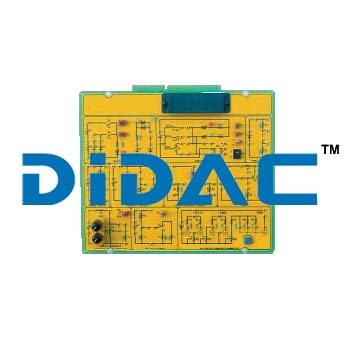0DC Fundamentals
Price And Quantity
• Pack/Packs
• 1
• , Pack/Packs
• 50000.00 - 500000.00 INR
• 100000 INR
Product Description

DC Fundamentals

Theoretical topic of DC Fundamentals

• DC power sources
• Batteries
• Conventional directions of voltages, and currents
• Ohms law
• Circuit with linear resistance and nonlinear resistance
• Series/parallel resistive circuits
• Power in dc circuits
• Linear/nonlinear variable resistor
• Voltage/current divider circuits
• Direct current meters
• Fault simulation

Circuit blocks of DC Fundamentals

• Batteries
• Switches
• Ohms law
• Series circuit
• Parallel circuit
• Series/Parallel circuit
• Power
• Linear/non Linear variable resistor
• Voltage divider
• Voltmeter/Ammeter/Ohmmeter

• 10 Per Month
• 10-30 Days
• Export Worthy
• ISO
Related Products